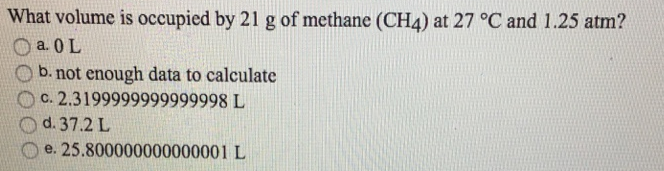# Problem: What volume is occupied by 21 g of methane (CH4) at 27°C and 1.25 atm?a. 0Lb. not enough data to calculatec. 2.3199999999999998 Ld. 37.2 L e. 25.800000000000001 L

###### FREE Expert Solution
82% (72 ratings)###### Problem Details

What volume is occupied by 21 g of methane (CH4) at 27°C and 1.25 atm?

a. 0L

b. not enough data to calculate

c. 2.3199999999999998 L

d. 37.2 L e. 25.800000000000001 L